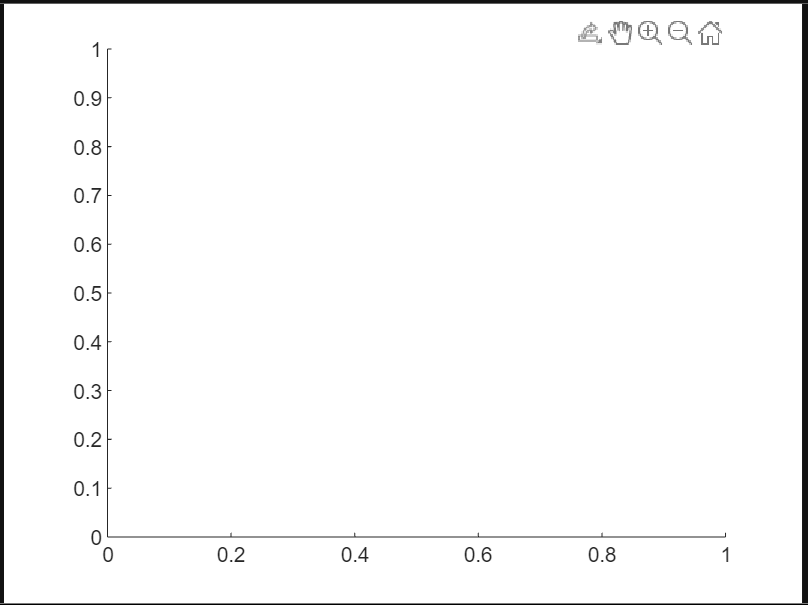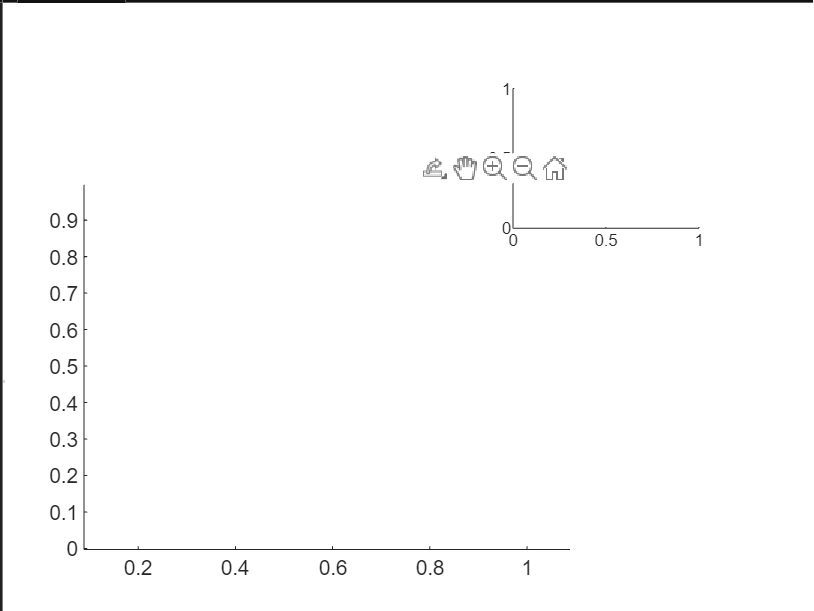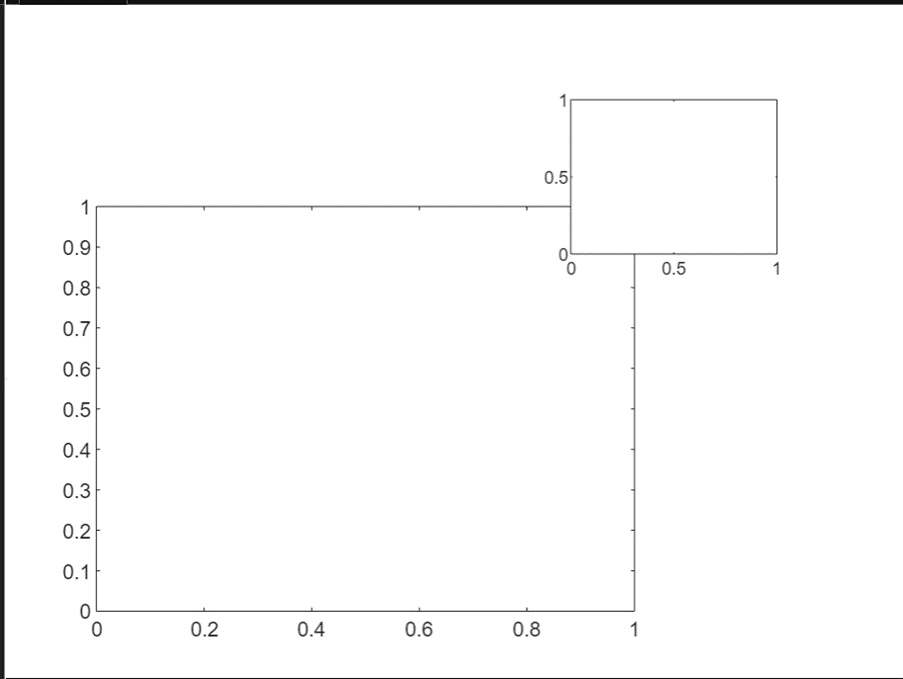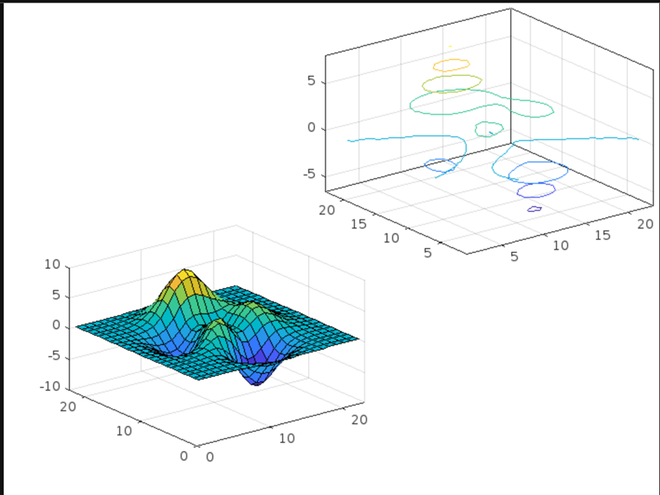GeeksforGeeks App
Open AppBrowser
Continue

# Create Cartesian Axes in MATLAB

By default, the cartesian axes are added to a figure in MATLAB when it is created as a graphical component however, MATLAB provides a function to do the particular job, the axes() function. This function creates cartesian axes in a figure. It is highly useful in cases where multiple cartesian planes are needed in a single figure component. Let us explore the function with examples.

### Syntax:

axes (parent, name, values)

• Parent – specifies the figure or class object where the axes are to be created.
• Name – gives that particular set of cartesian axes a specific name.
• Values – contain the behavioral values to define the axes

Let us create the default set of cartesian axes in a figure. Simply calling the axes without any arguments creates a default cartesian plane.

Example 1:

## Matlab

 `% MATLAB code for creating``% Cartesian Axes``axes`

Output:Creating multiple axes in a single figure involves the positioning of the second cartesian axes in the figure which can be done by the ‘Position’ argument. The syntax of axes function would then change to

axes_1 = axes (‘Position’, [<horizontal position of left bottom> <vertical position of left bottom> <height of axes> <width of axes>])

axes_2 = axes (‘Position’, [<horizontal position of left bottom> <vertical position of left bottom> <height of axes> <width of axes>])

Now, let us see an example to understand this.

Example 2:

## Matlab

 `% MATLAB code for ``% axes 1``axes1 = axes(``'Position'``,[0.1 0.1 0.6 0.6])`` ` `% axes 2``axes2 = axes(``'Position'``,[0.63 0.63 0.23 0.23])`

Output:Here, the axes one has its left bottom corner at [0.1 0.1] and its height and width are [0.6 0.6]. Similarly, the left bottom corner of axes2 is at [0.63 0.63] and its height and width are [0.23 0.23].

As can be seen in the above figure that it is hard to identify the boundaries of both plots. To resolve this, we can add boxes around each axis as follows.

Example 3:

## Matlab

 `% MATLAB code for add boxes around each axis``% axes 1``axes1 = axes(``'Position'``,[0.1 0.1 0.6 0.6],``'Box'``,``'on'``);`` ` `% axes 2``axes2 = axes(``'Position'``,[0.63 0.63 0.23 0.23],``'Box'``,``'on'``);`

Output:Now that we have seen different ways of creating cartesian axes, let us plot some dummy plots to see how it works. In doing so, you only need to specify the axes name as the first argument of any plotting function.

Example 4:

## Matlab

 `% MATLAB code for ``% Defining 2 axes``axes1 = axes(``'Position'``,[0.1 0.1 0.45 0.45],``'Box'``,``'on'``);``axes2 = axes(``'Position'``,[0.49 0.49 0.5 0.5],``'Box'``,``'on'``);`` ` `% Plotting in axes 1``surf(axes1,peaks(23))`` ` `% Plotting in axes 2``contour3(axes2,peaks(23))`

Output:My Personal Notes arrow_drop_up